# Annual Disability Statistics Compendium Standard Errors Companion 2023

## Introduction

Overview. New to the Collection this year (2023), the Annual Disability Statistics Standard Error Companion (referred to as the Companion) is designed for use alongside the Annual Disability Statistics Compendium (referred to as the Compendium) to understand the precision of data in applicable tables.

The Companion contains tables depicting standard error of frequency and SE of percent for tables that utilize American Community Survey Public Use Microdata Sample (ACS PUMS) data in the Compendium.

Who was the Companion designed for?

While primarily designed to support research and advocacy efforts, the Companion is available for anyone wanting to know the accuracy of the data in the Compendium. Knowing and communicating the accuracy of the data can bolster the impact of research, advocacy, and reports.

To use. Locate the table number in the top left corner of the page in the Compendium.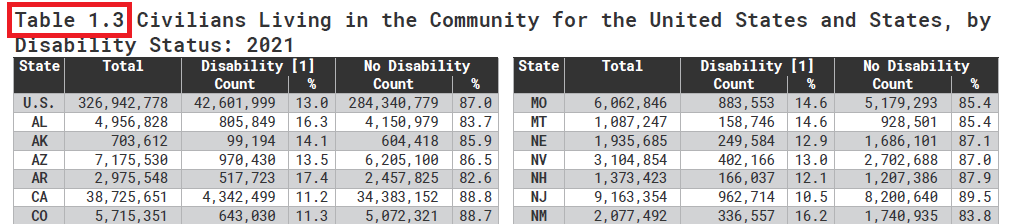Then locate the same table number in the Companion – it will have the abbreviation for standard error (SE) in front of the table number. If you cannot find the Compendium’s corresponding table in the Companion, that means the data used to produce the statistics in the Compendium was not ACS PUMS data.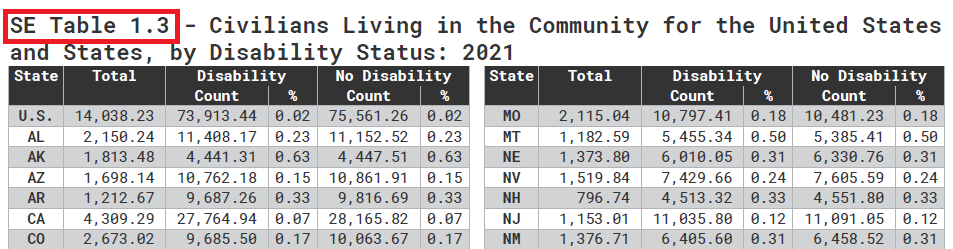Then compare the data from the Compendium to that in the Companion.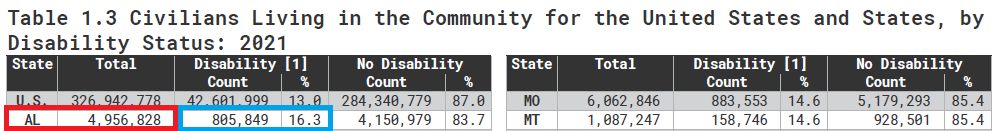Cross reference with same statistic in the Companion –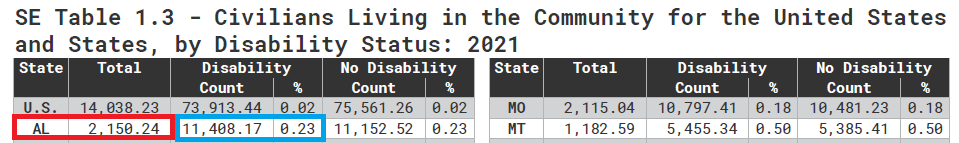Table 1.3 in the Compendium shows in 2021, Alabama had a total population of 4,956,828. The SE of frequency for the population of Alabama is 2,150.24.

Alabama’s total population with disability is reported in Table 1.3 as 805,849 people, or 16.3% of the population. The SE of frequency (see the Count column) is 11,408.17, and the SE of percent (see the % column) is 0.23%.

The lower the SE, the more likely the mean of the sample data is close to that of the population data.

What is Standard Error and why does the Companion only have SE tables for Compendium tables produced by ACS PUMS data?

Standard error (SE) is calculated for data sets that are produced by using a sample of the total population being studied – this is called sample data. Data produced through sampling is prone to bias because it is sourced from only a portion of the population being studied. Because the sample data has a slightly different data than the population data, the mean will be different than the mean of the population. The SE informs a data user how precise the mean of the sample data is, which, when coupled with a distributional assumption, can then be used to determine a confidence level. Confidence levels express the certainty by which the true population mean is contained within a specified bound (the confidence interval). ACS PUMS data along with data from surveys like the Behavioral Risk Factor Surveillance System (BRFSS) are sample data. This year we focused our efforts on producing SE tables to go along with tables in the Compendium that utilize ACS PUMS data.

Data sets that are produced using the entire population being studied – known as population data – do not have any sampling error because no subjects are excluded from the data. Therefore, we do not calculate SE for population data. Government agencies and programs that report administrative records are a form of population data. For example, the Social Security Administration (SSA) produces data that is based on the total count of people receiving benefits. Since the entire population of people receiving benefits from the SSA is included in the final data product, there is therefore no range of error in the data resulting from sampling. Standard error cannot be calculated for sections of the Compendium that utilize population data from the U.S. Department of Education’s Rehabilitation Services Administration (Vocational Rehab) and Individuals with Disabilities Education Act public data, the Veterans Benefits Administration, and the SSA.

Why is knowing the standard error important? How is it interpreted and what is it used for?

Standard error (SE) is a number or percent that represents the relative spread or uncertainty of the population mean. Its calculation specifies how accurately the sampled data demographically represents the total population. It is foundational to expressing the impact of statistics to the public.

In a simple random sample, standard error is calculated by dividing the sample’s estimated standard deviation by the square root of the sample size.

SE = σn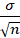Standard error is interpreted in the context of the data’s confidence interval (CI), is complemented by data’s margin of error (MOE), makes statistics understandable colloquially.

Confidence intervals are parameters produced by choosing a confidence level – typically 90-99% – then testing the data using the confidence level value. Confidence levels represent the certainty that the population mean – as if the entire population were included in the data – will fall within the confidence interval.

When using sample statistics, it is not possible to calculate an estimate that is 100% certain to match the (unknown) population estimate. But estimating the amount of error around our sample statistic will provide an opportunity to estimate a range of values (a confidence interval) that are likely to contain the true population value. Commonly, analysts will calculate confidence intervals that have confidence levels of between 95% and 99%, but sometimes researchers will use 90%. This means that one can expect the estimate to fall within the confidence interval 90-99% of the time – that is, if the population was resampled an infinite number of times. To calculate a confidence interval, standard errors are necessary.

Let’s say we’re 95% certain the results will fall within the confidence interval. First, calculate the significance level - which is the probability that the results will fall outside of the confidence interval. The significance level is represented by α.

100% - 95% = 5%

Then convert to decimal form and divide the result by two – this is because there are two ends of the normal distribution:

1. .95 = .5 ÷ 2 = .025

Significance level is typically expressed like this:

CL = 1- α

or:

1 = α + CL

Significance level (or α) is necessary to determine the critical value – a component in the calculation for the confidence interval and margin of error. Critical values are determined using z-scores, t-scores, or the chi-squared test among others, in combination with the significance level and – depending on the test – the degrees of freedom.

To calculate the confidence interval, add or subtract the sample mean (this varies from the population mean because studies typically do not include data from the whole population, but rather from a portion – or sample – of the population) from the product of the critical value (this is calculated using the confidence level) and the standard error.

CI = x̅ ±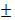CV σnThe margin of error is half the confidence interval. There are two sides (+ and -) of the sample mean in the normal distribution; the confidence interval is the total – from the negative side to the positive side, and the margin of error is only one side of the sample mean in the normal distribution. Therefore, the margin of error (MOE) equals the product of the critical value (CV) and the standard error (SE).

MOE = CV× SE

This is also expressed as:

MOE = CV × σn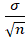What does this all mean?

As previously stated, standard error is interpreted in the context of the data’s confidence interval (CI), is complemented by data’s margin of error (MOE) and is understood as significant through the confidence level.

When applied to the Compendium, the standard errors in the Companion inform us of how close the sample mean is likely to the true population mean. It lets us know the precision of the data in the Compendium.